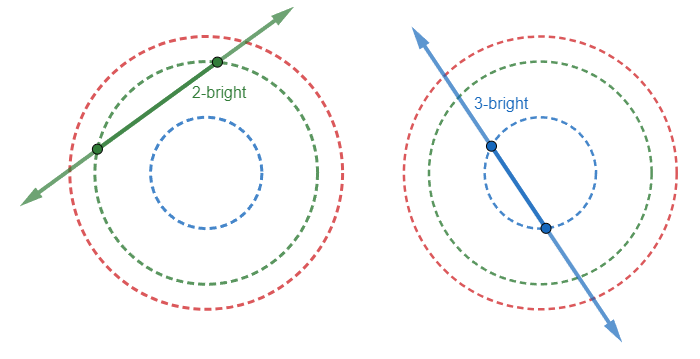# Schrödinger's BrightnessratingminusBattle of Brains 2023, Un...
Limits 1.5s, 512 MBAntman is in the quantum realm. He has $n$ orbits which are concentric and circular. These are called Schrödinger's orbits because whenever Antman observes orbit $i$, exactly two points at random on this orbit become photon sources and a light ray emerges connecting these two sources.

A ray of light is called $k$-bright if it comes in contact with exactly $k$ orbits.

It is 9:40 in the morning at CS427, and being late in statistics class Mahadi challenges Shamik with the following—

If Antman is currently observing orbit $i$, what is the probability that a $k$-bright light will emerge?

Shamik, not being a Marvel fan, claims the problem is so trivial that it is left as an exercise for the contestants.

## Input

The first line of input contains a single integer $n\;(1 \le n \le 10^5)$— the number of orbits.

The second line contains $n$ integers $r_1, r_2,…, r_n \;(1 \le r_i \le 10^9)$— where $r_i$ is the radius of orbit $i$.

The third line contains a single integer $q\;(1 \le q \le 5\cdot10^5)$— the number of queries to to answer.

Following each of the next $q$ lines contain two integers $i\;(1 \le i \le n)$and $k\;(0 \le k \le n)$— the orbit in observation and the desired brightness of the light.

It is guaranteed that all $r_i$ are distinct.

## Output

For each query, print the desired probability in a single line.

Your answer is considered correct if its absolute or relative error doesn’t exceed $10^{-6}$.

## Sample

InputOutput
3
239 77 169
2
2 3
1 1

1.000000000
0.499994427


There are infinitely many points in an orbit and there can be zero or infinitely many pairs of points that can produce a ray of light with our desired brightness.

### Submit